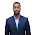January 09, 2018

Properties of Indifference Curves

January 09, 2018

An indifference curve shows various combinations of two goods that provides equal level of satisfaction to consumer. The Indifference curves are used to examine preferences of consumer. They are based on ordinal system such as first, second, third and so on. It means, if consumer likes mangoes more than oranges then he can give first rank to mangoes and second rank to oranges.  The table given below is illustrating the concept of indifference curve.

In the above indifference schedule, we can see there are five combinations of good X and Y. Each combination yields equal level of satisfaction to consumer. When these combinations are represented graphically and each point is joined together, the indifference curve is formed as shown below.

Properties of Indifference Curve

Following are the five most important properties or characteristics of indifference curves. Each property is illustrated with the help of a diagram.

1. Indifference Curve Slopes Downward
The indifference curve slopes downward from left to right. This is so because as consumer increases the consumption of x commodity, he has to scarify units of y commodity to maintain same level of satisfaction. Here, the law of marginal rate of substitution (MRS) applies which is the basis of indifference curve analysis. MRS is a rate at which units of one good can be substituted with units of another good, so that level of satisfaction remains same. This can be explained with the help of a diagram.

2. Higher Indifference Curve represents Higher Level of Satisfaction
Consumers have a preference for higher quantities. This is reflected in the indifference curves. Each indifference curve represents different level of satisfaction. The higher indifference curve provides a greater satisfaction than the lower curve because there are more units of X and Y commodity. In the given below diagram IC2 curve provides higher satisfaction to consumer than IC1. This is so because the consumer increases units of X and Y commodity.

3. Indifference Curves Cannot Intersect
The indifference curves cannot intersect. We have learnt that higher indifference curve provides higher satisfaction to consumer, so they cannot cut each other. In the given below diagram there are two indifference curves IC1 and IC2 intersecting each other. Since higher curve IC2 gives higher satisfaction to consumer it will never touch lower curve IC1.

4. Indifference Curve Cannot Touch Either Axis
Consumer has to buy combinations of two different commodities. He is not supposed to buy only one commodity. If the consumer buys only one commodity, the indifference curve will touch either x-axis or y-axis which is against the basic assumption of indifference curve. It can be seen from the given below graph that IC1 is touching y-axis at point OM which means consumer is consuming only Y commodity. Similarly, IC2 is touching x-axis at point ON which means consumer is consuming only X commodity.

5. Indifference Curves are Convex to Origin
Another property of indifference curves is that they are usually convex to the origin of their axis. This property is derived from the law of diminishing marginal rate of substitution. According to this law, as consumer increases consumption of X commodity and decreases consumption of Y commodity, the marginal rate of substitution of X for Y (MRSxy) goes on falling. Due to this relationship, the indifference curve has to be convex to its origin. The illustration is given below with the help of a diagram.

Share:

1.